Numerical Simulations

Researchers are making extensive use of the structural analysis platform, OpenSees, to simulate the nonlinear frame response to input ground motions.  This page reviews some of the analyses that have been performed up to this point in order to predict the behavior of this frame during the actual test.  The following figure depicts the nomenclature used in the results that follow.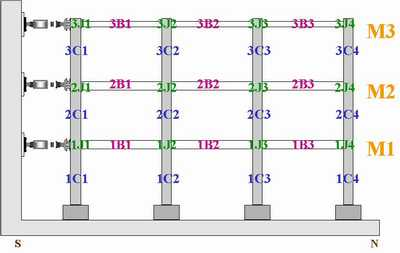Flexibility-based beam-column fiber elements, beam-column joint elements, and steel and concrete material models have been calibrated to data from subassembly tests of RC columns and composite beam-column connections conducted at NCREE earlier this year.

The RC Columns are modeled using OpenSees material models Concrete02 and Steel02 to represent the core/cover concrete and the reinforcing steel, respectively.  The following shows a comparison between the OpenSees model and an actual experiment (Tsai 2002).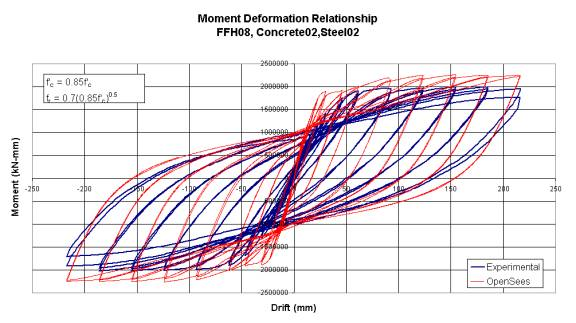The composite beams are also modeled using Steel02 and Concrete02 to represent the steel beam and the concrete slab.  The following shows a comparison of the OpenSees model and a test from Bursi/Ballerini (1996).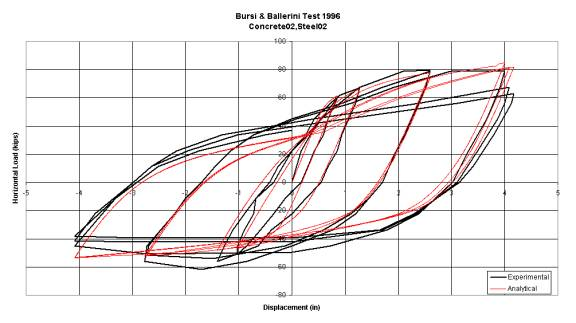The composite joints are modeled with a simple joint element that correctly models the kinematics of a finite joint and utilizes a nonlinear spring to represent the failure mechanisms.  The Steel02 and Hysteretic material models are used to represent this spring for both panel shear and bearing failure, respectively.  The following shows a comparison between the OpenSees model and an actual experiment (Kanno 1993).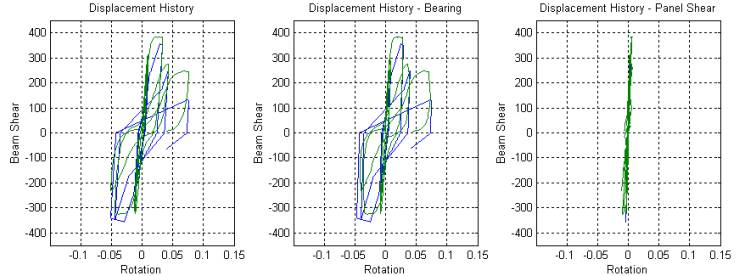The model includes a leaning column to represent the P-delta forces generated by the theoretical interior gravity frames.  This model has equal mass applied to each floor (M1=M2=M3=645kips).

The response spectrums of the two earthquakes, each scaled to a PGA of 1g, are shown in the following figures.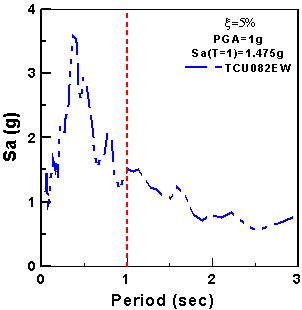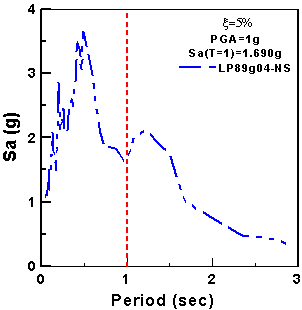# RESULTS

TCU082 – EW

50%in50year record scaled to a PGA of 0.275g

Equal Masses (M1=M2=M3=645kips)

1.

2.

3.

4.

LP89G04 – NS

10%in50year record scaled to a PGA of 0.427g

Equal Masses (M1=M2=M3=645kips)

1.

2.

3.

4.

TCU082 – EW

2%in50year record scaled to a PGA of 0.622g

Equal Masses (M1=M2=M3=645kips)

1.

2.

3.

4.

TEST SIMULATION

In order to simulate the actual testing conditions, we must subject the analytical model of the frame to the following four consecutive earthquakes:

1.       50%in50year TCU082-EW

2.       10%in50year LP89G04-NS

3.       2%in50year TCU082-EW

4.       10%in50year LP89G04-NS

Therefore each earthquake following the 1st record will start with a damaged structure and possibly some residual drift.  The results of these analyses are presented here.

EQ#1 - TCU082 – EW

50%in50year record scaled to a PGA of 0.275g

Equal Masses (M1=M2=M3=645kips)

5.

6.

7.

8.

EQ#2 - LP89G04 – NS

10%in50year record scaled to a PGA of 0.427g

Equal Masses (M1=M2=M3=645kips)

5.

6.

7.

8.

EQ#3 - TCU082 – EW

2%in50year record scaled to a PGA of 0.622g

Equal Masses (M1=M2=M3=645kips)

5.       Displacement and Story Shear Response

6.       RC Column Behavior

7.       Composite Beam Behavior

8.       Composite Joint Behavior

EQ#4 - LP89G04 – NS

10%in50year record scaled to a PGA of 0.427g

Equal Masses (M1=M2=M3=645kips)

9.       Displacement and Story Shear Response

10.   RC Column Behavior

11.   Composite Beam Behavior

12.   Composite Joint Behavior